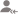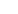true# Learn Introduction To Graphs with Free Lessons & Tips

Post a Lesson

Asked on 10 Jan Learn Introduction To Graphs

1. Draw the points (5, 4) and (4, 5). Do they represent the same point?

Asked on 10 Jan Learn Introduction To Graphs

2. Draw a line passing through (2, 1) and (1, 2). Find the coordinates of the points at which this line... read more
2. Draw a line passing through (2, 1) and (1, 2). Find the coordinates of the points at which this line meets the x-axis and y-axis. read less

Asked on 10 Jan Learn Introduction To Graphs

3. Draw the line passing through (2,3) and (3,2). Find the coordinates of the points at which this line... read more
3. Draw the line passing through (2,3) and (3,2). Find the coordinates of the points at which this line meets the x-axis and y-axis. read less

Asked on 10 Jan Learn Introduction To Graphs

.4: Plot the following points on a graph sheet. Verify if they lie on a line: (a) A(4,0), B(4, 2),C(4,6), D(4, 2.5)

Asked on 10 Jan Learn Introduction To Graphs

5. Plot the following points on a graph sheet. Verify if they lie on a line (b) P(1, 1), Q(2, 2), R(3,3), S(4, 4) "

Asked on 10 Jan Learn Introduction To Graphs

6.A bank gives 10% Simple Interest (S.I.) on deposits by senior citizens. Draw a graph to illustrate... read more
6.A bank gives 10% Simple Interest (S.I.) on deposits by senior citizens. Draw a graph to illustrate the relation between the sum deposited and simple interest earned. Find from your graph (a) the annual interest obtainable for an investment of ` 250. (b) the investment one has to make to get an annual simple interest of ` 70." read less

Asked on 10 Jan Learn Introduction To Graphs

6.State whether True or False. Correct that is false. (i) A point whose x coordinate is zero and y-coordinate... read more
6.State whether True or False. Correct that is false. (i) A point whose x coordinate is zero and y-coordinate is non-zero will lie on the y-axis. (ii) A point whose y coordinate is zero and x-coordinate is 5 will lie on y-axis. (iii) The coordinates of the origin are (0, 0) read less

Asked on 10 Jan Learn Introduction To Graphs

7. Plot the point (4, 3) on a graph sheet. Is it the same as the point (3, 4)? "

Asked on 10 Jan Learn Introduction To Graphs

9. Plot the following points and verify if they lie on a line. If they lie on a line, name it. A (1,... read more
9. Plot the following points and verify if they lie on a line. If they lie on a line, name it. A (1, 1), B (1, 2), C (1, 3), D (1, 4) read less

Asked on 10 Jan Learn Introduction To Graphs

10. Plot the following points and verify if they lie on a line. If they lie on a line, name it. (iii)... read more
10. Plot the following points and verify if they lie on a line. If they lie on a line, name it. (iii) K (1, 3), L (2, 3), M (3, 3), N (4, 3) read less

UrbanPro.com helps you to connect with the best in India. Post Your Requirement today and get connected.

Overview

Questions 11

Total Shares0 Followers
x

Please select a Tag

X

### Find Tutors, Trainers & Institutes near you

Post requirement and connect with the tutors in your locality

• Select the best Tutor
• Book & Attend a Free Demo
• Pay and start Learning### Want to learn something New?

Find best tutors, trainers & institutes near you on UrbanProUrbanPro.com is India's largest network of most trusted tutors and institutes. Over 55 lakh students rely on UrbanPro.com, to fulfill their learning requirements across 1,000+ categories. Using UrbanPro.com, parents, and students can compare multiple Tutors and Institutes and choose the one that best suits their requirements. More than 7.5 lakh verified Tutors and Institutes are helping millions of students every day and growing their tutoring business on UrbanPro.com. Whether you are looking for a tutor to learn mathematics, a German language trainer to brush up your German language skills or an institute to upgrade your IT skills, we have got the best selection of Tutors and Training Institutes for you. Read more